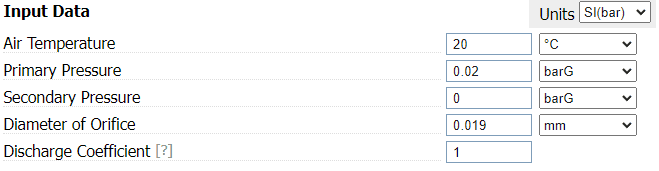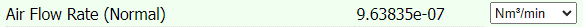# Find hole size for allowable leak in pressure vessel

bgaw
TL;DR Summary
Need to find the hole size for an an allowable leak in a small, low pressure vessel.
Hello, all. I am completely new here so please be gentle.

I have a small, cylindrical, sealed ("no leak") pressure vessel with an approximate volume of 27 cm3. It has a constant supply of air pressure at 8"WC. I'm trying to figure out how big of a hole it can have if there is an allowable leak/flow rate of 1.00 sccm.

I feel like this isn't that hard of a problem and I should probably know how to do it, but alas I've been out or school for awhile and I do not. And I haven't been able to find anything in my internet searches that seem to tackle this problem. Most of what I can find deals with water and the Bernoulli equation, but I don't think that applies here. I'm pretty sure I'm missing some information/data. I'm not necessarily looking for an exact answer, but mostly just how to do this as I may need to do other versions of this in the future. Thank you in advance.

Welcome to PF.
How long is the hole, how thick is the cylinder wall ?
What shape is the hole ?

Homework Helper
Haello @bgaw ,##\qquad ##!​
.. to find anything in my internet searches
Funny, a search for 'orifice air flow' yields a lot of useful stuff ! Even a calculator, but perhaps it's more useful to look through the equations to see if they apply for your range of ##\Delta p##

##\ ##

bgaw
Welcome to PF.
How long is the hole, how thick is the cylinder wall ?
What shape is the hole ?
The wall thickness is 0.06". And the hole will be round.

Homework Helper
Expanding: google search for 'vapour leak flow' has this in fourth place, with some formulas on page 8/9.

Incidentally, the calculator gives me a worst case initial guess of 19 ##\mu\textsf {m}##:Where I am assuming sccm is 10-6 Nm3/min

##\ ##

Homework Helper
The wall thickness is 0.06". And the hole will be round.
So the 19 ##\mu##m is ##<<## 1500 ##\mu##m (0.06") and you want to include a pressure drop over the narrow channel ...

##\ ##

FluidDroog
I understand the Problem as follows:
• The sealed volume is estimated to have a leak of 1 sccm of Air.
• The leak flow channel is assumed circular.
• The upstream pressure of the flow channel is 8"water column (8psig = 156.5 kPaA).
• The donstream pressure of the flow channel is 0" water column (0 psig = 101.325 kPaA).
• The temperature of the system is isothermal at T= 22 deg. Celsius (295.15 Kelvin)
The goal is:
What is the diameter, D, of a flow channel if the channel length, L, is 0.06"?

Solution:
First I would use Poiseuille, equation #3 from reference . For this you will need approximations for the viscosity and molecular weight of an Air mixture, which can be found using free software CoolProp .
Molecular weight = 2.897E-02 kg/mol and viscosity = 1.831E-05 Pa*s.

$$mdot =\frac{ \pi * M * R^4 * (P_1^2 - P2^2)}{16*\mu*L*R*T}$$
$$sccm = mdot * 60e3 * V_m / M$$
Where Vm is the standard molar volume defined in NIST codata .
By substitution and algebraic re-organization. The Diameter is found to be:
D = 23.38 micrometers

The next step is to identify if Poiseuille is accurate at these conditions. Poiseuille assumes incompressible, which means the Mach# < 0.3 . To do that, we need the speed of sound for Air, roughly 345 m/s.
$$Mach = \frac {velocity} {speed of sound}$$

Where the velocity of the gas is found from
$$velocity = \frac {mdot}{Area * Density}$$
Where density is calculated at the average pressure in the channel, 1.516 kg/m3 

I estimate the Mach # to be 0.1, thus Poiseuille is accurate and the problem is finished. This number agrees well with BvU's answer of 19 micron. The diameter is larger due to the friction along the micro channel.
If the Mach # was large, then BvU's recommendation for Orifice flow calculator or other equations would need to be considered.

References:
 Calibration of laminar flow meter for process gases. https://tsapps.nist.gov/publication...8560&msclkid=c048ffcdac0711ecae5f6f6526f549c4
 [Link spam redacted by the Mentors]
 Standard Molar Volume https://physics.nist.gov/cgi-bin/cuu/Value?mvolstd|search_for=molar+volume
 Compressible Flow https://en.wikipedia.org/wiki/Compressible_flow?msclkid=867dcdb2ac0911ec9db4f69c0927b111

Last edited by a moderator:
•cjl